# 4 Donation

#eval=FALSE because this is already done in Main

source(here("code", "modeling_functions.R"))

source(here::here("build","labelling_eas.R")) # needs to have been run also -- some of these objects are used below
# Folder to save plots in
plots_folder <- here("analysis", "plots", "donations_20")

Note on feedback (unfold)

I’d love to get your feedback on this report. It’s in the ‘bookdown’ format produced through Rmd, (with folded code as well as some other folding supplemental bits in case you are curious, but these could be ignored)

Written by me with heavy input from Oska Fentem and guidance from David Moss and others (including Peter Wildeford and Nik Vetr).

Much/most of this will be input into an EA Forum post, but that post may leave out some of the more technical and detailed content, and then refer/link to this hosted report (embedded with the other reports).

(Most) margin notes will become footnotes in the forum post. Folding boxes will mainly be dropped in the forum version.

Ideally, you could leave your feedback right in the web site using the Hypothes.is tool (in our private group; see thread, but public is OK too).

If you have difficulties with that, or with any part of this format, please let me know. Of course I also appreciate feedback in any form, including in Slack, a document, on the Github repo, etc.

I hope this work may be relevant even beyond this specific context, and thus would love loads of feedback because

• I think represents somewhat of a change in how we’re addressing the EA survey (e.g., the cross-year analysis)
• because I/we hope to apply some of these methods and formats to other projects and other data in the movement building, EA messaging, and fundraising space!

Thanks so much!!

## 4.1 Introduction and summary

Charitable donation (and earning-to-give) has been, and continues to be a prominent, prevalent, and impactful component of the Effective Altruism movement. The EA Survey has been distributed between 2014 and 2020, at roughly 15 month intervals. As a result, surveys were released at various points in the year, ranging from April to August, and no survey was released in 2016. In each survey we asked EAs about their charitable donations in the previous year, and their predicted donations for the year of the survey. Our work in this post/section reports on the 2020 survey (2019 giving), but our analysis extends to all the years of the EA survey.

In this post (and the accompanying ‘bookdown’ supplement chapter), we consider donation responses, presenting both raw numbers, and descriptive, predictive, and causally-suggestive analysis. We present simple numbers, statistical comparisons, vizualisations, and descriptive and ‘predictive’ (machine learning) models. We cover a range of topics and concerns, including:

Our modeling work work considers how donations (total, share-of-income, and ‘donated over 1000 USD’) jointly relates to a range of characteristics. We first present ‘descriptive’ results focusing on a key set of observable features of interest, particularly demographics, employment and careers, and the ‘continuous features’ age, time-in-EA, income, and year of survey. We next fit ‘predictive’, allowing the ‘machine learning’ models themselves to choose which features seem to be most important for predicting donations.

* Note (to put in EA Forum post only): A ‘dynamic version’ of this document (an R-markdown/Bookdown), with folded code, margin notes, some interactive graphs and tables, and some additional details, can be found here. This may be helpful for anyone that wants to dig into this more deeply, and perhaps for those who are data, code, and statistics-inclined.
In the narrative below, we simply refer to “donations” rather than “reported donations” for brevity. Unless otherwise mentioned, all figures simply add, average, or otherwise summarize individual responses from the EA Survey years mentioned.

Programmers note: Most/many numbers included in the text below are soft-coded, and thus can automatically adjust to future data or adapted data. However, where we cite previous posts, these numbers are largely hand-coded from previous work.

require(scales)

#can also move stuff to plotting_functions.R

# Define breaks and limits
breaks <- c(0, 10^(1:10))
max_lim <- max(filter(eas_all, !year %in% c(2014, 2015))[c("donation_usd", "donation_plan_usd")], na.rm=TRUE)
density_breaks <- seq(0, 1, 0.2)[-1]

# Define same parameters for x and y axis
scales <- list(limits = c(0, max_lim), trans = scales::pseudo_log_trans(base=10),
breaks = breaks,
labels = scales::label_number_si(prefix = "$"), expand=c(0,0)) scatter_theme <- theme_minimal() donate_charity_names <- eas_20 %>% dplyr::select(matches("donate_")) %>% dplyr::select(-matches("action_|_later")) %>% names() don_tot_freq <- eas_20 %>% summarise(across(c(all_of(donate_charity_names)), ~sum(as.numeric(.x) > 0, na.rm = TRUE))) %>% slice(1) %>% unlist(., use.names=TRUE) dev_health_chars <- c("donate_deworm_the_world_c", "donate_givewell_c", "donate_schistosomiasis_control_c", "donate_give_directly_c", "donate_against_malaria_found_c", "donate_global_health_develop_c") animal_chars <- c("donate_mercy_for_animals_c", "donate_humane_league_c", "donate_ea_animal_welfare_fund_c", "donate_good_food_institute_c", "donate_ace_c") ea_meta_chars <- c("donate_rethink_charity_c", "donate_80k_c", "donate_cea_c", "donate_ea_foundation_c", "donate_ea_meta_fund_c") lt_ai_chars <- c("donate_machine_intelligence_c", "donate_long_term_future_fund_c") other_chars <- c("donate_center_applied_rational_c", "donate_global_health_develop_c", "donate_other1_c", "donate_other2_c", "donate_other3_c", "donate_other4_c", "donate_other5_c") all_chars <- c(dev_health_chars, animal_chars, ea_meta_chars, lt_ai_chars, other_chars) #all_char_labels <- list(animal_don = "Animal welfare", dev_don = "Global health + development", ea_meta_don = "EA meta and organization", lt_ai_don="Long term & AI", other_don = "Other" ) -- moved to #all_char_labels2 <- list(dev_don = "Global health + development", animal_don = "Animal welfare", ea_meta_don = "EA meta and organization", lt_ai_don="Long term & AI", other_don = "Other" ) -- moved to #here::here("build","labelling_eas.R") # moved to build side: # eas_20 <- eas_20 %>% sjlabelled::var_labels(all_char_labels) count_notna <- function(x) sum(!is.na(x)) where_don_dummies <- c("d_dev_don", "d_animal_don", "d_ea_meta_don", "d_lt_ai_don", "d_other_don") # Construct charity-specific aggregations (?move to build side) where_don_vars <- c("dev_don", "animal_don", "ea_meta_don", "lt_ai_don", "other_don") eas_20 <- eas_20 %>% mutate( num_named_dons = rowSums(!is.na(select(., one_of(all_chars)))), dev_don = rowSums(across(all_of(dev_health_chars)), na.rm = TRUE), d_dev_don = dev_don > 0, animal_don = rowSums(across(all_of(animal_chars)), na.rm = TRUE), d_animal_don = animal_don>0, ea_meta_don = rowSums(across(all_of(ea_meta_chars)), na.rm = TRUE), d_ea_meta_don = ea_meta_don>0, lt_ai_don = rowSums(across(all_of(lt_ai_chars)), na.rm = TRUE), d_lt_ai_don = lt_ai_don>0, other_don = rowSums(across(all_of(other_chars)), na.rm = TRUE), d_other_don = other_don>0 ) %>% mutate_at(.vars =where_don_vars, funs(ifelse(num_named_dons==0, NA, .)) ) eas_20 %<>% labelled::set_variable_labels(.labels = as.list(all_char_labels), .strict=FALSE) pct_tot <- function(x) { x/NROW(eas_20)*100 } num_don <- sum(eas_20$donation_2019_c>0, na.rm=TRUE)
num_na_don <- sum(is.na(eas_20$donation_2019_c)) zero_don <- sum(eas_20$donation_2019_c==0, na.rm=TRUE)

tot_don <- sum(eas_20$donation_2019_c, na.rm=TRUE) #for all years, for USA nonstudents only tot_don_all_usa <- sum(eas_all$donation_usd[eas_all$d_live_usa==1 & eas_all$d_student==0], na.rm=TRUE)

tot_inc_all_usa <- sum(eas_all$income_c_imp_bc5k[eas_all$d_live_usa==1 & eas_all$d_student==0], na.rm=TRUE) tot_share_don_us_nonstudent <- tot_don_all_usa/tot_inc_all_usa tot_don_dev <- sum(eas_20$dev_don, na.rm=TRUE)
tot_don_animal <- sum(eas_20$animal_don, na.rm=TRUE) tot_don_ea_meta <- sum(eas_20$ea_meta_don, na.rm=TRUE)
tot_don_lt_ai <- sum(eas_20$lt_ai_don, na.rm=TRUE) med_don <- median(eas_20$donation_2019_c, na.rm=TRUE)
mean_don <- mean(eas_20$donation_2019_c, na.rm=TRUE) mean_don_not_new <- mean(eas_20$donation_2019_c[eas_20$year_involved_n!=year_s], na.rm=TRUE) mean_don_18 <- mean(eas_all$donation_usd[eas_all$year==2019], na.rm=TRUE) mean_don_18_not_new <- mean(eas_all$donation_usd[eas_all$year==2019 & eas_all$year_involved!="2019"], na.rm=TRUE)

plan_donate_2019_c <- filter(eas_all, year == 2019) %>% pull(donation_plan_usd)

mean_plan_18_19 <- mean(plan_donate_2019_c, na.rm=TRUE)
med_plan_18_19 <- median(plan_donate_2019_c, na.rm=TRUE)

med_not_new <- median(eas_20$donation_2019_c[eas_20$year_involved_n!=year_s], na.rm=TRUE)

top_1p3don <- eas_20 %>% select(donation_2019_c) %>% slice_max(donation_2019_c, prop =.013) %>% sum()
top_1p3share <- top_1p3don/tot_don

### 4.1.1 Summary (some key results and numbers)

• 55.5% of EAs in the 2020 survey reported making a charitable donation in 2019, 13.7% reported making zero donations, and 30.8% did not respond to this question. (Thus, of those who responded, 80.3% reported making a donation in the prior year.)

• Participants reported total donations of 10,695,926 USD in 2019 (cf 16.1M USD in 2018).

• However, the number of survey participants has declined somewhat, from 2509 in 2019 (1704 of whom answered the donation question) to 2056 (1423 answering the donation question) in 2020.*

• Over the past years, we see no strong trend in median or mean donation amounts reported.

* All figures here refer to survey responses, so we won’t write ‘reported in the survey’ each time. These (2019-20) numbers exclude a single survey donation response in the billions that was ruled to be implausible. A total of 2 observations were dropped for implausible income, donations, or ages. Averages are for those who answered the donation question(s), including those who reported donating zero. Nonresponses are not counted in these statistics except where specifically mentioned. Unless otherwise mentioned, all figures simply add, average or otherwise summarize individual responses from the EA Survey years mentioned.

• The median annual donation in 2019 was 528 USD (cf 683.92 USD in 2018).

• The mean (reported) annual donation for 2019 was 7,516 USD (cf 9,370 for 2018) or 8,607 USD excluding those who joined in 2020 (cf 10,246 USD for 2018 excluding those who joined in 2019).

• The median annual donation in 2019 excluding those who joined EA in 2020 was 761 USD (cf. 990 USD for the comparable median for 2018/2019 and 832 USD for 2017/2018). (See ’donation and income trends in EA’ for more details).

• In 2019 1.3% of donors accounted for $6,437,404 in donations or 60% of the survey total. (Cf in 2018 1.3% of donors accounted for 57% of donations.) med_don_share <- median(eas_20$don_share_inc_19, na.rm = TRUE)
med_don_share_imp_bc <- median(eas_20$don_share_inc_19_imp_bc5k, na.rm = TRUE) earn_filter <- quos(d_student==0, income_c>10000) med_don_share_imp_ns_10k <- eas_20 %>% filter(!!!earn_filter) %>% summarise(med=median(don_share_inc_19, na.rm = TRUE)) tot_inc <- sum(eas_20$income_c, na.rm=TRUE)

tot_inc_imp_bc <- sum(eas_20$income_c_imp_bc5k, na.rm=TRUE) share_don_gt_10pct <- sum(eas_20$don_share_inc_19>=.1, na.rm = TRUE)/sum(!is.na(eas_20$don_share_inc_19)) share_don_gt_10pct_imp <- sum(eas_20$don_share_inc_19_imp_bc5k>=.1, na.rm = TRUE)/sum(!is.na(eas_20$don_share_inc_19_imp_bc5k)) share_don_gt_5pct_imp <- sum(eas_20$don_share_inc_19_imp_bc5k>=.05, na.rm = TRUE)/sum(!is.na(eas_20$don_share_inc_19_imp_bc5k)) share_don_gt_10pct_earn <- eas_20 %>% filter(!!!earn_filter) %>% transmute(share_don_gt_10pct = sum(don_share_inc_19>=.1, na.rm = TRUE)/sum(!is.na(don_share_inc_19)) ) %>% unlist %>% . #don gt 10pct ... by gender #eas_20 %>% # mutate(d_don_gte10_imp = don_share_inc_19_imp>=.1) %>% # tabyl(gender_manual, d_don_gte10_imp) %>% tabylstuff() • The median percentage of income donated in 2019 was 2.96% (cf 3.23% in 2018). • However, if we impute “0 and missing incomes” at “group medians for student-status and country,”* the median percentage of income donated was 2% for 2019. * Many respondents do not reveal their income, or report zero or implausibly small incomes (if we consider income to include transfers and family support); among these, many do report donations. To get a meaningful measure of average shares of income donated (and other stats) including these individuals, we need to put some measure reflecting yearly spending power in the denominator. We thus make a rough imputation, selecting the average income for individuals from their same country and same student-status who do report an income value. To avoid sensitivity to outliers, countries with small numbers of participants are lumped together into an “other” group for this imputation. Where this (or reported income) falls below 5000 USD, we ‘bottom-code’ it this as at 5000 USD. (Note that we hope to improve this imputation in future work, incorporating features such as age in the imputation.) • Mean share of total (imputed) income donated was 9.44% (imputing income where below 5k or missing) or 12.5% without imputation. • 20% of EAs who answered the donation question reported donating 10% or more of their income in 2019 (if we impute income; otherwise 25.6% without imputation; this compares to 20% in 2018, without imputation). • The median percent of income donated by full-time-employed non-students who earned more than$10,000 was 2.92%, and of this group 23.9% donated 10% of their income or more in 2019 (cf 3.38% and 24% in 2018).

• Overall, those taking the EA survey tend to report donating a substantially greater share of income than those in the general US population – (web link).

pct_don <- function(x) {
sum(don_tot_freq[x])/sum(don_tot_freq)*100
}

pct_ddon <- function(x) {
op(
sum(x != 0, na.rm=TRUE)/sum(notNA(x), na.rm=TRUE)*100
)
}
don_stats <- eas_20 %>%
filter(num_named_dons>0) %>%
select(all_of(where_don_vars)) %>%
vtable::sumtable(
summ=c('notNA(x)', 'sum(x != 0)', 'sum(x != 0)/notNA(x)', 'mean(x)', 'sd(x)', 'pctile(x)', 'pctile(x)'),
summ.names = c('Number of Responses', 'Number reporting donation to cause', 'Share of reporters donating to cause', "Mean donation of reporters (including 0's)", 'Sd', "Median", "90th pct"),
digits=c(0,0,2,0,0,0,0),
simple.kable = TRUE,
labels = all_char_labels2,  #it's a horrible workaround but we need to have the order of these the same as the table order ... I think it's a flaw of sumtable
title = "Donations by category (where indicated)",
out="kable") %>%
kable_styling()

#todo (low-priority) -- replace with .summ hijacked command

n_rep_char <- sum(eas_20$num_named_dons>0, na.rm=TRUE) don_stats_by_gwwc <- eas_20 %>% mutate(GWWC Pledge = case_when( action_gwwc==1 ~ "Yes", action_gwwc==0 ~ "No" )) %>% filter(num_named_dons>0) %>% select(all_of(where_don_vars), GWWC Pledge) %>% vtable::sumtable(group = "GWWC Pledge", group.test=TRUE, summ=c('notNA(x)','sum(x != 0)/notNA(x)', 'mean(x)', 'sqrt(var(x)/length(x))', 'pctile(x)'), summ.names = c('N Responses', 'Share positive', 'Mean', "Median"), digits=c(0,2, 0,0,0), simple.kable = TRUE, labels = all_char_labels2, #it's a horrible workaround but we need to have the order of these the same as the table order ... I think it's a flaw of sumtable title = "Donations by category (where indicated), by GWWC", out="kable") %>% row_spec(1:1, bold = TRUE) %>% kable_styling() ddon_stats_by_gwwc <- eas_20 %>% mutate(GWWC Pledge = case_when( action_gwwc==1 ~ "Yes", action_gwwc==0 ~ "No" )) %>% filter(num_named_dons>0) %>% select(all_of(where_don_dummies), GWWC Pledge) %>% vtable::sumtable(group = "GWWC Pledge", group.test=TRUE, summ=c('notNA(x)','sum(x != 0)/notNA(x)'), summ.names = c('N Responses', 'Donated to... ?'), digits=c(0,2), simple.kable = TRUE, labels = all_char_labels2, #it's a horrible workaround but we need to have the order of these the same as the table order ... I think it's a flaw of sumtable title = "Binary: Indicated donating to category, by GWWC", out="kable") %>% row_spec(1:1, bold = TRUE) %>% kable_styling() # .kable() %>% # .kable_styling("striped") #todo (low-priority) -- replace with .summ hijacked command • While 69.2% of respondents answered the donation question, only 20.9% answered at least one question about where they donated. • Among these, the charity that the most EAs stated that they donated to was the Against Malaria Foundation (AMF), with 122 reported donations (out of a total of 1462 reported donations). • Global Poverty charities continue to attract the largest counts and amounts of donations. 62% of those who answered the relevant question reported donating to this category. 26.9% of the total ‘where donated’ reports were to global poverty charities. We sum 1,703,870 USD in total donations reported as specifically going to global poverty charities. • This compares to 27.3% reporting donating, 10.5% of donations and $\$ 645,086 total donated for animal charities, • 17.2%, 5.81% and $\$ 330,910 for EA movement/meta charities, • and 18.2%, 5.61% and $\$ 418,403 for long term and AI charities, respectively. • Evidence is mixed on whether EAs’ donations in a year tend to exceed or fall short of the amount they planned to donate (as they reported in previous surveys). For the small share that can be tracked across years, donations tend to exceed plans (by around 60 USD at median, but over 1000 USD at mean). However, the overall distribution of donations for a particular year (including all respondents) tends to fall short of the distribution of planned donations (by about 450 USD at median and over 2000 at mean). • While at median EAs tend to report planning to donate the same amount this next year that they donate in each particular year, the average (mean) plan for next year is significantly larger. • Our descriptive models basically find that:* • age, being in a named ‘top EA’ big city, having taken the GWWC pledge, and an Earning-to-Give career are positively associated with donations, • while being ‘not employed’ (and to a lesser extent non-male gender and student status are negatively associated with this; • donation are roughly proportionally associated with income (approximately ‘unit elastic’), • as well as with age and ‘time in EA’ (with elasticities around 0.54 to 0.63, respectively). • Our predictive (ML) models highlight the importance of income and (to a lesser extent) age (each positively related to donation incidence and amounts). • These models perform moderately well, particularly in predicting ‘whether donated 1k USD or more’ (here it attains about 74% accuracy compared to 54% accuracy from simply ‘guessing the most common outcome’). * Caveat: not all of these coefficients are statistically significant by standard metrics. These results are ‘statistically stronger’ for our model of ‘whether donated 1000 USD or more.’ ### Why does the EA Survey ask about donations? What does it tell us? What is the “theory of change” for how learning about donation behavior will improve outcomes? We present some reasons why this may be useful:* * This should be considered a medium-run project: we will not be able to address all of these questions in the current post. • The magnitude of EAs’ donations informs ‘how much weight can we throw around’ in asking charities etc to appeal to us as a community? While we have other measures (discussed below) of the overall amounts and largest donations, the EA Survey conveys additional information about the donations of ‘large groups of moderate-income people who explicitly identify with EA.’ • This may offer insight into ‘what motivates and impedes donation behavior.’ • Donation behavior may be seen as one measure of EA engagement; our evidence may thus offer insight into ‘what motivates engagement.’ • Observing changes in donation patterns across time may alert us to potential problems and important changes in priorities, values, and the nature of the EA movement. Being able to predict future donation behavior may also help EA organizations better anticipate, budget, and plan (in conjunction with their existing data and models). • Predicting and describing typical donation rates can inform decisions like “which EAs seem likely to have more impact if they choose to go into direct work versus earning-to-give.”* * My impression is that previous work on ‘should I work directly for good or earn-to-give’ has tended to focus on earning potential, presuming that those who are in large amounts will donate at a certain planned rate. However, an equally important question may be "what share or amount of income should we expect people who pursue earning-to-give to end up donating? This question seems particularly important in the presence of value drift. (However, one might argue that the individual’s own understanding of his or her future behavior might dominate, and not be easily integrated with the insight that we gain from the broad predictions using survey data.) • Perhaps more controversially (and we are raising this idea but not promoting it), EAs’ donation amounts might be seen as incentive-compatible ‘votes’ telling us what people in the movement want the EA movement to focus on? However, note people need not be truthfully reporting here, so if we allow for mis-statement, this is far from incentive compatible. ## Total EA donations, magnitudes in context Considering the magnitude of the donations… The$10,695,926 USD in donations reported above seems likely to be a small share of total EA-affiliated giving, perhaps less than 1/4 of the total (excluding the super-rich and institutional givers), perhaps even a far smaller share (see extrapolations below).

Previous estimates suggest that, even among very highly-engaged EAs, only about 40% complete the EA survey. While we might assume that people with a lower ‘cost of time’ (and, all equal, lower incomes) are likely to be over-represented in the EA survey, those who donate more might be more likely to respond to these particular questions. Other estimates suggest that only about 20% of GWWC members complete the survey. As noted above, only 69.2% of survey respondents answered the ‘past year donation’ question in 2020. We present some extrapolations below, our own and others.

Even within the survey, the largest mass of donations are heavily concentrated among a few givers. We expect that the distribution of donations in EA overall is even more heavily skewed, with large donors and foundations (such as Tuna and Moskowitz of Open Philanthropy accounting for a lion’s share. The table below uses data from Open Phil’s Grants database, divided by year and cause area).*

effectivealtruismdata.com provide further interesting visualizations of the magnitude, sources, and recipients of EA donations.

library(scales)

research_terms <- "research|univ|study|UC|trial|scholar|fellow|macreoeconomic|rethink|study|feasibility|analysis|evaluation"

focus_area_names <- c(
Criminal Justice Reform = "Crime/Justice",
Farm Animal Welfare = "Farm Animal",
Global Health & Development = "Glob. Health/Dev.",
Scientific Research = "Scient. Res.",
Potential Risks from Advanced Artificial Intelligence = "AI risk",
Biosecurity and Pandemic Preparedness = "Biosec.",
Other areas = "Other",
Macroeconomic Stabilization Policy = "Macro-econ",
Global Catastrophic Risks = "Glob. Catastr. Risk",
Immigration Policy = "Immig. Policy",
Land Use Reform = "Land Ref.",
U.S. Policy = "US policy",
History of Philanthropy = "Hist. of Phil."
)

as_tibble() %>%
mutate(
amount = as.numeric(gsub('[$,]', '', Amount)), amount_usd_k = amount/1000, date = lubridate::my(Date), year = lubridate::year(date), focus_area = dplyr::recode(Focus.Area, !!!focus_area_names), focus_area = as.factor(focus_area)) %>% select(-Amount, -Date) ( op_res_grants_tab_yr_area <- open_phil_grants %>% dplyr::group_by(year, focus_area) %>% # drop_na(!!yvar, !!treatvar) %>% summarise(total = sum(amount_usd_k, na.rm = TRUE)) %>% spread(year, total, fill=0) %>% adorn_totals("row") %>% adorn_rounding(digits = 0) %>% arrange(-2020) %>% rename_with(~snakecase::to_sentence_case(.)) %>% # Change focus_area to Focus Area .kable(caption = "Open Philanthropy grants by year and area, in$1000 USD",
col.names = NA) %>%
row_spec(1:1, bold = TRUE) %>%
.kable_styling("striped")
)
Table 4.1: Open Philanthropy grants by year and area, in $1000 USD Focus area 2012 2013 2014 2015 2016 2017 2018 2019 2020 2021 Total 3,100 3,045 29,978 34,627 135,666 312,480 197,047 297,963 273,776 146,992 Glob. Health/Dev. 2,000 2,550 22,134 26,971 66,054 124,217 75,105 40,706 101,671 32,788 Scient. Res. 0 0 0 0 9,039 47,550 25,160 53,860 67,203 3,163 Biosec. 0 0 0 300 5,323 28,841 9,388 21,566 26,468 9,580 Farm Animal 0 0 0 0 14,436 27,957 27,977 39,870 25,057 14,319 AI risk 0 0 0 1,186 6,564 43,222 4,160 63,244 15,847 62,961 Other 1,100 0 1,300 210 2,872 6,144 18,140 13,018 14,677 11,098 Crime/Justice 0 445 3,000 1,141 24,591 21,421 20,375 55,522 10,534 2,537 Glob. Catastr. Risk 0 0 0 500 3,170 9,118 12,901 1,803 5,701 4,622 Immig. Policy 0 0 2,780 915 1,324 1,800 400 1,785 3,700 0 Macro-econ 0 0 435 2,179 1,906 1,405 2,450 3,150 2,317 5,323 Land Ref. 0 0 0 773 387 640 890 3,440 600 600 Hist. of Phil. 0 50 25 2 0 166 0 0 0 0 US policy 0 0 303 450 0 0 100 0 0 0 Extrapolations and further benchmarks: • Ben Todd’s recent post estimates that the EA community is donating$420 million per year, which “has grown maybe about 21% per year since 2015,” and “around 60% was through Open Philanthropy, 20% through other GiveWell donors, and 20% from everyone else.”

• A recent post by tylermaule estimates $263 million in ‘funding ’global funding of EA causes.’* * This relies on Open Phil’s Grants Database, GiveWell’s Metrics Report, EA funds intake figures, and Animal Charity Evaluators’ Metrics report. • Giving What We Can reports roughly$70 million in donations per year, in recent years.**

** At end of Dec. 2020 they reported that their roughly 5000 members “donated more than $203,443,730”to highly effective charities". In December 2019 the comparable figure was 126.8 million, suggesting that roughly 77 million was donated in a single year. However, the same figure:$25,309,348, was listed both in December of 2018 and 2017, so the figures may not be constantly updated. GWWC gives data on the destinations of ‘GWWC donations that go through EA funds.’

• GiveWell reported “GiveWell donors contributed over 150 million to our recommended charities in 2019.” Of course, the above large donations/grant totals may not all be coming from donors aligned with EA, and may not entirely go towards the most effective charities. The donations also may not be well-described by the donations recorded in the EA survey. in the fold/footnote, we consider the importance of EA-aligned donations in comparison to non-EA donations to similar causes. We return to this in a supplemental appendix section (web link), specifically focusing on US nonstudents, comparing these to results from a national survey. A further question is whether the few hundreds of millions of dollars in EA-aligned donations is substantial in comparison to non-EA donations to similar causes (e.g., developmentaid “Top trends in private philanthropic donations for development” reports the OECD figure of7.8 Billion in private philanthropic donations for development in 2018, and 200-300 billion in total charitable donations per year from the USA alone.)

Some quick responses:

1. Naturally, we anticipate EA donations will tend to be much more effective per dollar, perhaps orders of magnitude more so. (A basic case, with some references, is given here. However, Tomasik and others present credible arguments for being skeptical of the claims of vast differences in effectiveness within a given domain.)

2. Even if EA donations were small in relation to global giving, they still have an important impact, and this is the domain we can control. (Relatedly, we should not fall victim to the ‘drop in the bucket’ and ‘proportion dominance’ biases in considering this.)

3. “Where, when, and how much EAs are giving” may be an important informative measure of beliefs and priorities (discussed further below).

extrap_tot_ea_don <- (tot_don +
tot_don*0.5 *
(num_na_don/(num_don+zero_don))) / 0.3

Our own rough extrapolations suggest, perhaps very conservatively, $43.6 million USD could be a reasonable central guess for the total amount of annual donations coming from non-billionaire EAs, i.e., the sort of EAs who respond to the EAS.* * This extrapolation simply multiplies the reported$10,695,926 USD by 1 + 0.445 and divides by 2, to adjust for the share of respondents who did not answer this question, presuming they give at half the rate of those who do answer. Next we divide by 0.3, averaging the 20% and 40% estimates of EA survey nonresponse noted above. I presume that billionaire EAs are extremely unlikely to complete the survey or report their total donations in this form. Implicitly, we assume respondents are reporting accurately. This extrapolation should not be taken too seriously. David Moss has taken this one step further, with a brief ‘Fermi estimate’ in Guesstimate making the uncertainty over each parameter explicit, and expressing a confidence/credible interval with midpoint 78 million and 95% bounds 41-140 million USD.

## 4.2 Career paths: Earning-to-give

Although there may have been a recent decline in earning-to-give (ETG), it continues to be a popular career path. (We discuss career paths further in the EA Survey 2020: Demographics post under ‘Careers and education’*)

* In an earlier version of that post, the “which of the following best describes your current career” result had been misstated, showing the share of responses to this multi-response question rather than the share of individuals selecting ETG.

“We were requested to change the question between 2018/2019 and 2020… but looking at non-students (who are largely already in their careers), the responses across years may still be comparable and appear to show a slight decline in E2G”

In the tables and graphs below, the apparent steep drop in the number indicating ETG from the 2019 to the 2020 survey seems likely to be overstated (as a result of a requested change in the question language and options provided).*

* Question texts

2018: “What broad career path are you planning to follow?” [4 options]

2019: “If you had to guess, which broad career path(s) are you planning to follow?” [9 options]

2020: “Which of the following best describes your current career?” [11 options]

Note that the changing composition of EA survey respondents may also affect this.

Still, the responses for non-students might be less sensitive to the changes in the survey question as they are more likely to be in a career path as their ‘current career.’ These responses also suggest some decline in EtG.

etg_rates_all <- eas_all %>%
filter(year>2014) %>%
group_by(year) %>%
summarise( "Count" = n(),
"Share ETG" = mean(as.numeric(d_career_etg))
)

etg_rates_ns <- eas_all %>%
filter(year>2014) %>%
filter(d_student==0) %>%
group_by(year) %>%
summarise( "Count" = n(),
"Share ETG" = mean(as.numeric(d_career_etg))
)

(
etg_rates_tab <- bind_cols(etg_rates_all, etg_rates_ns[-1]) %>%
magrittr::set_names(c("Year", "All responses", "Share EtG", "Nonstudents", "Nonstudents: Share EtG")) %>%
kable(caption = "Rates of 'Earning-to-give' by year and student status (see caveats)", digits=3) %>%
.kable_styling()
)
Table 4.2: Rates of ‘Earning-to-give’ by year and student status (see caveats)
Year All responses Share EtG Nonstudents Nonstudents: Share EtG
2015 2362 0.217 980 0.322
2017 1845 0.220 671 0.380
2018 2599 0.303 1791 0.336
2019 2509 0.283 1898 0.262
2020 2056 0.151 1035 0.232
  #     tabyl(year, d_career_etg) %>%
#     tabylstuff_nocol(cap = "Non-students only; Rates of 'Earning-to-give' (see caveat)")
#

# (
# etg_rates_ns <- eas_all %>%
#     filter(d_student==0) %>%
#     tabyl(year, d_career_etg) %>%
#     tabylstuff_nocol(cap = "Non-students only; Rates of 'Earning-to-give' (see caveat)")
# )

# (
#   etg_rates_tab <- eas_all %>%
#   group_by(year, d_student) %>%
#   filter(!is.na(d_student)) %>%
#   summarise( "Count" = n(),
#              "Share ETG" = mean(as.numeric(d_career_etg))
#              ) %>%
#   pivot_wider(names_from =d_student,
#               values_from=c(Count, "Share ETG")
#               ) %>%
#   set_names(c("Year", "Nonstudents", "Students", "Nonstudents: Share EtG", "Students: Share EtG")) %>%
#   kable() %>%
#     .kable_styling()
#)

#todo - medium priority: combine the above tables into a single table: overall, just for students  with just n,
(etg_rates_plot <- eas_all %>%
group_by(year, d_student) %>%
filter(year>2014) %>%
filter(!is.na(d_student)) %>%

#@oska (low-med priority todo): we should functionalize these mutations for computing se and CIs (or find someone who has done). We do it again and again, and the code is bulky
#maybe incorporate my se_bin function
#@oska todo ... also functionalize or otherwise preserve a good version of this graph

# Calculate standard error, confidence bands and change student factor levels
summarise(
m_etg = mean(as.numeric(d_career_etg)),
se = se_bin(d_career_etg)) %>%
mutate(
etg_low = m_etg - 1.96*se,
etg_high = m_etg + 1.96*se,
d_student = as.factor(if_else(d_student == 0, "Non-student", "Student")),
year = as.factor(year)) %>%

ggplot(aes(x=year, y=m_etg, colour = d_student, group = d_student))  +
geom_pointrange(aes(ymin = etg_low,
ymax = etg_high),
position = position_dodge(width=0.5)) + # Ensure that bars don't overlap
geom_line(position = position_dodge(width=0.5)) +
xlab("Mean (and 95% CI) response share in 'Earning-to-give'") +
ylab("Share of sample") +
scale_color_discrete("") + # Remove legend title
scale_y_continuous(labels = scales::percent_format(accuracy = 1L), limits=c(0,NA), oob = scales::squish) + # Change y-axis to percentages
theme(legend.position = c(0.9, 0.95),
#legend.background = element_rect(fill=alpha('blue', 0.001)),
legend.key = element_blank())
)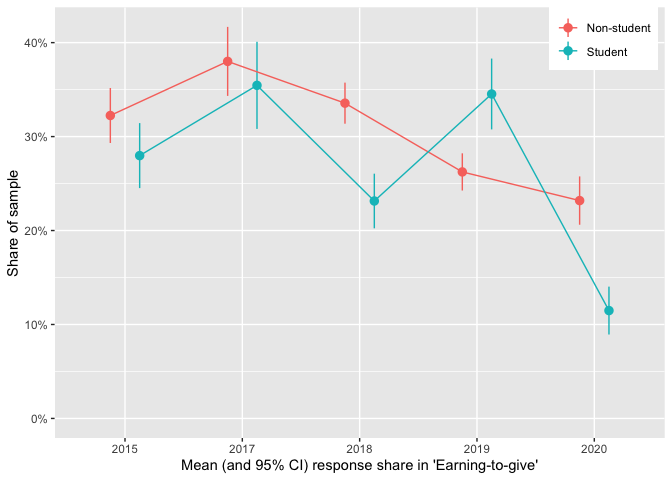The decline in ETG is less dramatic among non-students (over 23% of non-student respondents still report ETG as their ‘current career’), but it nonetheless appears to be fairly strong and consistent from 2017-present.*

* We do not include 2014 in the above tables and plots because of very low response rates to the student status and EtG-relevant questions.

## 4.3 Donation totals: descriptives

Note, we report on amounts ‘planning saved in 2020 to donate later in an appendix section.’

### Overall donations, totals by groups

Below, we present a histogram of positive reported 2019 donations by all respondents. Note that:

• the horizontal axis is on a logarithmic scale,
• 13.7% of the 2,056 total respondents reported donating zero, and
• 30.8% of the total respondents did not report their donation amount.
• As noted above, we will often simply refer to ‘donations’ rather than ‘reported donations,’ for brevity.
eas_20$don_19_p1 <- as.numeric(eas_20$donation_2019_c+1)
#adapting from EA survey 2019 Rscript_analysis.md

donation_2019_c <- eas_20$donation_2019_c require(scales) don_breaks <- c(50, 100, 200, 300, 500, 1000, 2500, 5000, 10000, 25000, 50000, 100000, 250000, 500000, 1000000, 2500000) eas_20 %<>% rowwise() %>% mutate(donation_2019_c_50 = max(donation_2019_c, 50)) %>% ungroup ( donhist_19 <- eas_20 %>% hist_plot_lscale(eas_20$donation_2019_c_50, breaks = don_breaks) +
geom_vline_mean(donation_2019_c) +
geom_vline_med(donation_2019_c) +
geom_vline_90(donation_2019_c) +
labs(title="Histogram of 2019 Donations", x="2019 $Donations (bottom-coded at 50)", y = "Number of respondents") )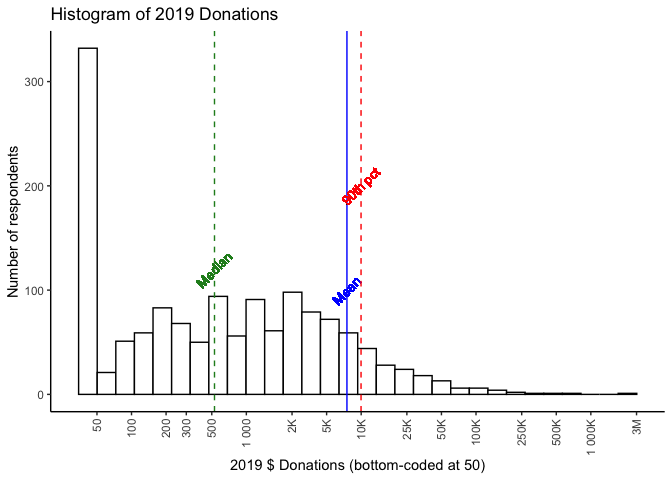# Todo (medium importance): Overlay a display of 'overall percentage shares' ... so we know where the 80th and 90th percentile are, etc. In 2019 we reported: a donation of 1000 USD per year … would place one in the top half of EA donors (specifically, the 55th percentile), whereas being in the top 10% of donors would require donating 11,000 USD and the top 1% 110,000 USD. The results for 2020 (for 2019 donations) are comparable; the median donation (of those reporting) is 528 USD, a donation of$1000 puts you in the 59.5th percentile. Being in the top 10% requires donating 9,972 and being in the top 1% means donating 89,560 USD.

As in previous years, the mean far exceeds the median, (and falls close to the 90th percentile!); a very small number of very large donations dwarf the size of most others. We illustrate this in the ‘treemap’ plot below, which divides the total reported contributions into groups by size-of-contribution.

require(treemapify)

geom_treemap_opts <- list(treemapify::geom_treemap(alpha = 0.7),
geom_treemap_text(fontface = "italic", colour = "white", place = "centre",
grow = TRUE, min.size = 1 ),
theme(legend.position = "none",
plot.title = element_text(hjust = 0.5))
)

(
don_share_by_size <- eas_20 %>% select(donation_2019_c, donation_2019_c_split) %>%
group_by(donation_2019_c_split) %>%
summarise(total_don = sum(donation_2019_c, na.rm=TRUE)) %>%
mutate(don_share = round(total_don/sum(total_don)*100)) %>%
filter(!is.na(donation_2019_c_split)) %>%
ggplot(aes(area = total_don, fill= donation_2019_c_split,
# Include percentage of total donation
label = paste(donation_2019_c_split, paste0(don_share, "%"), sep = "\n"))) +
geom_treemap_opts +
ggtitle("Share of total 2019 donation amount, by donation size")
)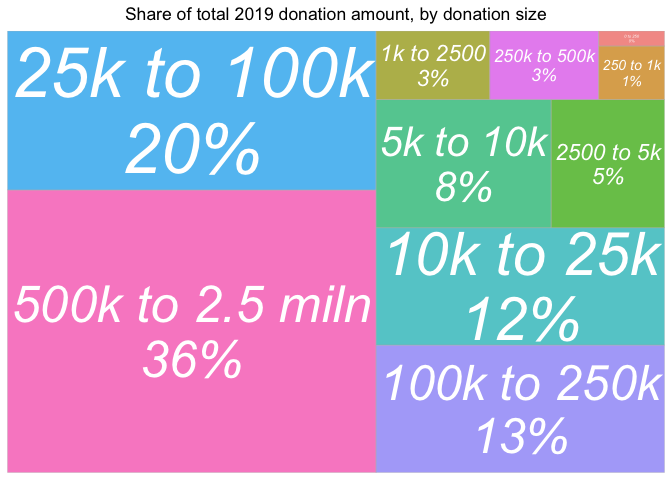Over a third of total reported contributions reported for 2019 come from contributions over 500,000 USD, with another 20% coming from contributions between 25k and 100k. Contributions of under 2500 USD represent less than 5% of the total.

Next we consider ‘which career paths are driving total donation totals?’; mapping the share of total 2019 donations similarly, accompanied by a table of their overall shares of respondents, for comparison.*

* This figure excludes 486 participants who provided no response to the career question, 0.236 of the sample. These participants reported a total of 2,766,310 in donations which makes up 25.9% of the total reported donations for 2019.

#library(treemapify)

(
don_by_career <- eas_20 %>% select(career_, donation_2019_c) %>%
group_by(career_) %>%
filter(!is.na(career_)) %>%
summarise(total_don = sum(donation_2019_c, na.rm=TRUE),
n = n()) %>%
mutate(don_share = round(total_don/sum(total_don)*100),
freq = n/sum(!is.na(eas_20career_)) ) %>% ggplot(aes(area = total_don , fill=freq, # Include percentage of total donation label = paste(career_, paste0(don_share, "%"), paste0("(Pop:", round(freq*100) , "%)"), sep = "\n"))) + geom_treemap_opts + # theme(legend.position = "bottom") + #todo -- add title to legend explaining that it's the survey pop; get better colors for this scale_fill_continuous(name = "Frequency", label = scales::percent, trans = "reverse") + labs(title= "Share of 2019 donations by career path", subtitle = "(Share of survey population in parentheses; darker = larger share)") )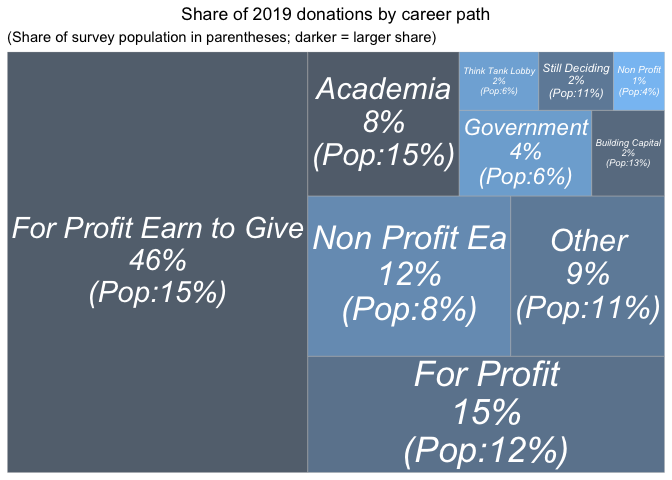career_tab <- eas_20 %>% mutate(Career = na_if(career_, "na")) %>% filter(!is.na(Career)) %>% tabyl_ow_plus(Career, caption="Shares in each career path", title_case = TRUE) #Todo: the right column needs to be x100 or say 'share' instead of 'percent' Those reporting ‘for profit-earning to give’ career paths represent the largest share, nearly half of the total donations, despite making up only about 15% of the sample (of those answering this question). Those with ‘for profit’ careers who do not say they are earning to give donate about 15% of the total, roughly in proportion to their 12% share of the sample. However all of these differences may reflect differences in income and wealth levels, as well as differences in underlying characteristics of people who choose different career paths. Direct work does not seem to be obviously coming at the expense of donations. Those pursuing careers working at EA-affiliated non-profits account for a somewhat higher share of donations (12%) than their (8%) share of the sample. (However, we do not know how much these particular EAs would have given had they chosen a different career.) Obviously, income levels are different between these career paths. We put this in perspective in the plot below. grp_sum <- function(df, xvar, yvar, groupvar) { df %>% dplyr::select({{xvar}}, {{yvar}}, {{groupvar}}) %>% group_by({{groupvar}}) %>% drop_na({{xvar}}, {{yvar}}, {{groupvar}}) %>% summarise( mn_y = mean({{yvar}}), mn_x = mean({{xvar}}), med_y = median({{yvar}}), med_x = median({{xvar}}), se_y = sd({{yvar}}, na.rm=TRUE)/sqrt(length({{yvar}})), se_x = sd({{xvar}}, na.rm=TRUE)/sqrt(length({{xvar}})) ) %>% group_by({{groupvar}}) %>% # Calculate confidence intervals mutate( lower = max(0, mn_y - 1.96*se_y), upper = mn_y + 1.96*se_y ) } plot_grp <- function(df, groupvar, labsize=4, labangle=90, force = 1, fp = 1, mo=10, bp=1, arrow=NULL) { df %>% ggplot(aes(x=mn_x, y=mn_y, label = {{groupvar}})) + geom_point() + geom_abline(intercept = 0, slope = 0.1, colour="violetred1") + geom_smooth(method=lm, alpha=0.7) + geom_errorbar(aes(ymin = lower, ymax = upper), alpha=0.7) + scale_y_continuous( oob = scales::squish) + scale_x_continuous( oob = scales::squish) + ggrepel::geom_text_repel( size = labsize, angle = labangle, max.overlaps=mo, force=1, force_pull = fp, box.padding = bp, arrow = arrow, color="brown", alpha=0.75) } ( don_inc_career_plot <- eas_20 %>% mutate(Career = na_if(career_, "na")) %>% filter(!is.na(Career)) %>% grp_sum(income_c_imp_bc5k, donation_2019_c, Career) %>% plot_grp(Career, labsize=3) + xlab("Mean income in USD (imputed if <5k/missing)") + ylab("Mean donations, CIs") + scale_y_continuous(limits=c(-10000, 30000), oob = scales::squish) )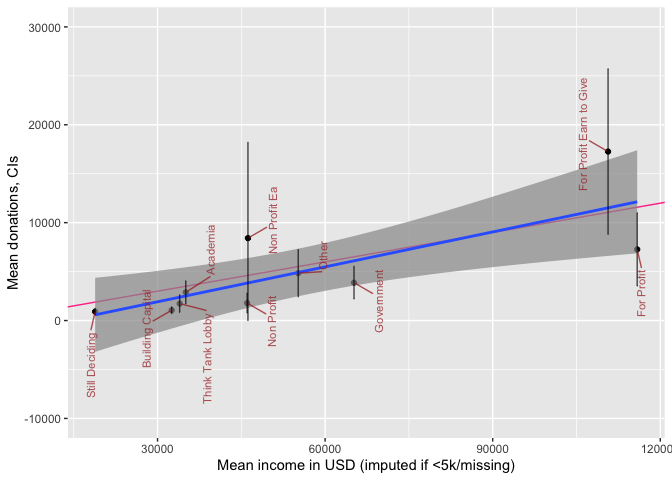The plot above depicts mean income and mean donations by ‘career group,’ with 95% CI’s for the latter. We superimpose a ‘line of best fit’ (blue, with smoothed 95% intervals for this rough fit) and a ‘10% of income donation’ line (red). Unsurprisingly, for-profit ‘not-EtG’ are below the fitted line, and ‘for-profit EtG’ above this line, although 95% CIs are fairly wide. We also note that among people in non-profit careers, there are similar average incomes whether or not the non-profit is EA-aligned, but the non-profit EA people seem to donate somewhat more (although the CI’s do overlap). Next, we present reported donation amounts by income groupings (imputing income where missing or below 5000 USD).* * However, the figure does remove observations where income as well one of either country or student status is missing, thus income cannot be simply imputed from these. #p_load(treemapify) ( don_share_by_income <- eas_20 %>% select(donation_2019_c, income_c_imp_bc_k, income_c_imp_split) %>% filter(!is.na(income_c_imp_bc_k)) %>% group_by(income_c_imp_split) %>% summarise(total_don = sum(donation_2019_c, na.rm=TRUE), n = n()) %>% mutate(don_share = round(total_don/sum(total_don)*100), freq = n/sum(!is.na(eas_20income_c_imp_split))) %>%

ggplot(aes(area = total_don, fill= freq,
# Include percentage of total donation
label = paste(income_c_imp_split,
paste0(don_share, "%"),
paste0("(Pop:", (round(freq*100, 1)) , "%)"),
sep = "\n"))) +
geom_treemap_opts +
scale_fill_continuous(name = "Frequency",
label = scales::percent, trans = "reverse") +
labs(title= "Share of 2019 donations by income groups",  subtitle = "(Share of survey population in parentheses; darker = larger share)")
)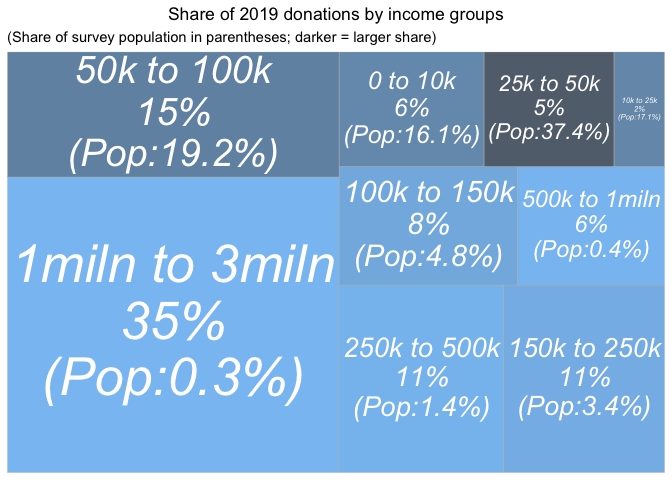earn_tab <- eas_20 %>%
tabyl_ow_plus(income_c_imp_split)

Compare the above graph to the ‘donations by donations size’ graph.

The largest earners (the 6 people earning 1 million USD or more) represent 35% of the donations (cf the largest donors represent 36% of the donations). However, the second-highest earners, the 8 people earning between 500k and 1 million USD represent only 6% of the donations (cf 20% from the second-highest donation group). In fact, the second largest share of total 2020 donations come from the second-largest (in population) income-group in our sample, the 395 people earning between 50K and 100K USD.

Finally, we report donation totals by country.

First for 2019 donations alone:

#p_load(treemapify)

(
don_share_country <- eas_20 %>% select(donation_2019_c, country_big) %>%
group_by(country_big) %>%
summarise(total_don = sum(donation_2019_c, na.rm=TRUE),
n = n()) %>%
mutate(don_share = round(total_don/sum(total_don)*100),
freq = n/sum(!is.na(eas_20$country))) %>% ungroup() %>% filter(don_share != 0 & !is.na(country_big)) %>% ggplot(aes(area = total_don, fill= freq, # Include percentage of total donation label = paste(country_big, paste0(don_share, "%"), paste0("(Pop:", op(round(freq*100, 0)) , "%)"), sep = "\n"))) + geom_treemap_opts + #scale_fill_continuous(name = "Frequency", label = scales::percent, trans = "reverse") + scale_fill_continuous(name = "Frequency", label = scales::percent, trans = "reverse") + labs(title= "Share of 2019 donations by country", subtitle = "(Share of survey population in parentheses; darker = larger share)") )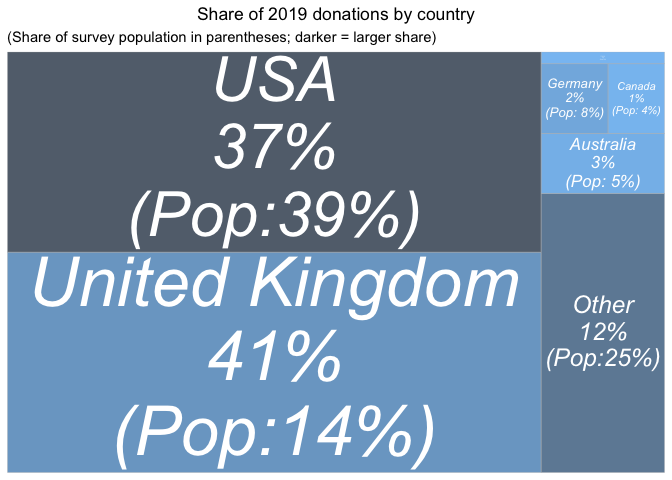#; darker = larger share Next, pooling across all years of the EA survey (without any weighting or adjustment): ( don_share_country_all_years <- eas_all %>% select(donation_usd, country, year) %>% filter(!is.na(country)) %>% group_by(country) %>% summarise(total_don = sum(donation_usd, na.rm=TRUE), n = n()) %>% ungroup() %>% mutate(don_share = round(total_don/sum(total_don)*100), freq = n/sum(!is.na(eas_all$country))) %>%

filter(don_share > 0.1) %>%
mutate(country = snakecase::to_title_case(country)) %>%
ggplot(aes(area = total_don, fill= freq,
# Include percentage of total donation
label = paste(country,
paste0(don_share, "%"),
paste0("(Pop:", op(round(freq*100, 0)) , "%)"),
sep = "\n"))) +
geom_treemap_opts +
scale_fill_continuous(name = "Frequency",
label = scales::percent, trans = "reverse") +
theme(legend.position = "none",
plot.title = element_text(hjust = 0.5)) +
labs(title= "Share of total (all years) donation amounts by country", subtitle = "(Share of survey population in parentheses; darker = larger share)")
)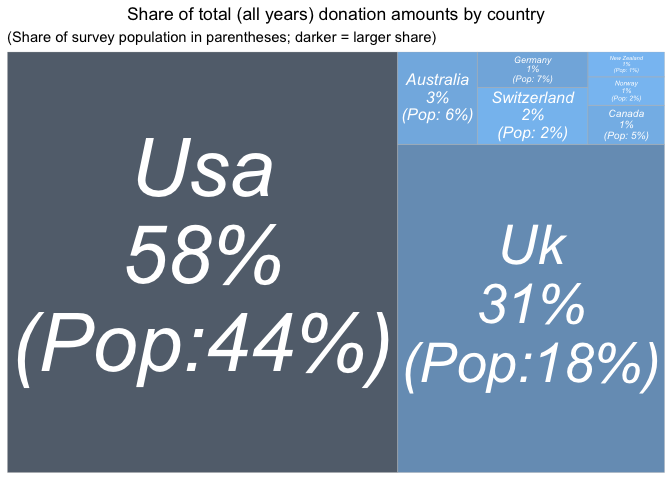And again, ‘Winsorizing’ donations at 100K USD (setting larger donations at this value), to reduce the impact of outliers:

(
don_share_country_all_years_w <- eas_all %>% select(donation_usd, country, year) %>%
filter(!is.na(country)) %>%
rowwise() %>%
mutate(donation_usd_w = min(donation_usd, 100000)) %>%
ungroup() %>%
group_by(country) %>%

summarise(total_don_w = sum(donation_usd_w, na.rm=TRUE),
n = n()) %>%
ungroup() %>%
mutate(don_share = round(total_don_w/sum(total_don_w)*100),
freq = n/sum(!is.na(eas_all$country))) %>% filter(don_share > 0.1) %>% mutate(country = snakecase::to_title_case(country)) %>% ggplot(aes(area = total_don_w, fill= freq, # Include percentage of total donation label = paste(country, paste0(don_share, "%"), paste0("(Pop:", op(round(freq*100, 0)) , "%)"), sep = "\n"))) + geom_treemap_opts + scale_fill_continuous(name = "Frequency", label = scales::percent, trans = "reverse") + theme(legend.position = "none", plot.title = element_text(hjust = 0.5)) + labs(title= "Share of 100k-Winsorised donations by country; all years", subtitle = "(Share of survey population in parentheses; darker = larger share)") )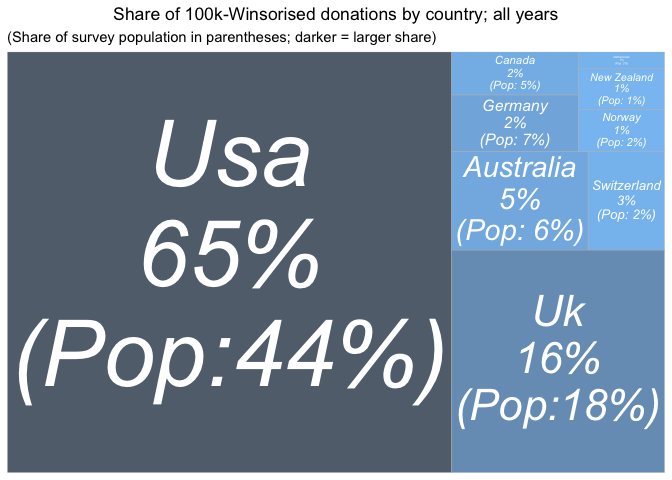#TODO - @oska -- UK and USA in all-caps above We report the shares (0-1) of the total survey population coming from each country below: #TODO - @oska -- capitalization below #TODO - @oska -- sort by shares below ( country_tab <- eas_all %>% group_by(country_big) %>% filter(year>2014) %>% mutate( year_2020 = case_when( year==2020 ~ "2019 share.", TRUE ~ "pre-2019 share." ), Country = str_to_title(country_big), ) %>% tabyl(Country, year_2020) %>% adorn_percentages("col") %>% .kable(digits=2, caption="Shares (0-1) of survey population by country; larger countries only", label=TRUE) %>% .kable_styling() ) Table 4.3: Shares (0-1) of survey population by country; larger countries only Country 2019 share. pre-2019 share. Australia 0.04 0.05 Canada 0.03 0.04 Czech Republic 0.02 0.00 France 0.02 0.01 Germany 0.06 0.05 Netherlands 0.02 0.01 New Zealand 0.02 0.01 Norway 0.02 0.01 Other 0.11 0.06 Sweden 0.01 0.01 Switzerland 0.02 0.02 Uk 0.11 0.13 Usa 0.30 0.32 NA 0.22 0.29 and we give a year-by-year animation of the shares of donations from each country: #d_anim <- "Y" #library(gganimate) anim_filename <- here(plots_folder, "animated_tree_plot.gif") if (exists("d_anim")) { if (d_anim == "Y") { animated_dons_country <- eas_all %>% select(year, donation_usd, country_big) %>% group_by(year, country_big) %>% filter(year>2014) %>% summarise(total_don = sum(donation_usd, na.rm=TRUE)) %>% mutate(don_share = round(total_don/sum(total_don)*100)) %>% ggplot(aes(area = total_don, fill= country_big, # Include percentage of total donation label = paste(country_big, paste0(don_share, "%"), sep = "\n"))) + geom_treemap_opts + ggtitle("Share of total 2019 reported donation amounts by country") anim <- animated_dons_country + transition_states(year, state_length = 3) + ggtitle("Share of total {closest_state} reported donation amounts by country") gganimate::anim_save(anim_filename, anim) anim } else{ knitr::include_graphics(anim_filename) } } if (!exists("d_anim")){ knitr::include_graphics(anim_filename) }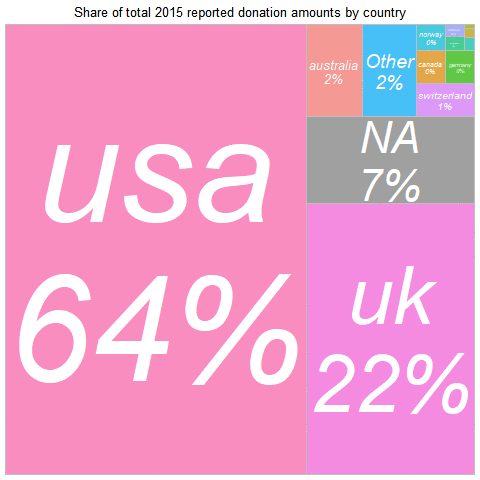#Todo (medium importance): slo In 2019, the largest summed donation amount came from • the UK (about 11% of the sample but 41% of the donations) • and the USA (30% of the sample and 37% of the donations). Across all years: • the USA represents the largest amount of donations, • with the UK a close second, Again, the UK ‘punches far above its weight.’ Note that the UK share may be understated, if UK donors claim the matching ‘Gift Aid’ but do not report it as part of their donation.* * In the UK the government’s ‘Gift Aid’ policy supplements all reported donations made by UK taxpayers by an additional 25%. Again, these raw difference may reflect differences in income and life circumstances among survey respondents from different countries. The outsized UK share also seems to be driven by a few large outlying donations – when we Winsorise donations at 100K USD, the UK no longer overperforms. We have shown ‘where the donations were in 2019’ (and across years). However, we are not suggesting that this provides direct evidence of differences in EA generosity by country. We return to presenting a ‘controlled descriptive picture’ in our modeling work. ### 4.3.1 Donation (shares) versus income and GWWC pledge We also looked at the percentages of pre-tax income that EAs were donating, based on the 1,563 EAs who disclosed both income and donation data. As in previous years, most EAs were donating significantly less than the 10% Giving What We Can Pledge… However, as the graph below shows, there is a marked ‘bump’ in the donors giving at around the 10% figure, perhaps due to the Giving What We Can Pledge target around this amount, or due to the figure’s wider popularity as a target (e.g. in tithing). Below, we depict donations as a share of income. The histograms below are first only for those with positive reported incomes, and next with the previously discussed income imputation. The blue vertical line depicts the share of total (imputed) income donated by all respondents, with the green line depicting the median and the red line the 90th percentile. These plots show similar patterns as in 2018. scale_x_set <- list(scale_x_continuous(limits=c(0,0.35), n.breaks=20)) ( don_share_inc_19_hist <- eas_20 %>% hist_plot(don_share_inc_19) + geom_vline_med(eas_20$don_share_inc_19, tgap=0.01) +
geom_vline_mean(tot_don/tot_inc, tgap=0.01, label = "Overall share") +
geom_vline_90(eas_20$don_share_inc_19, tgap=0.005) + scale_x_set + labs(title="2019 Donations/Income (no imputing)", x="2019 Donations/income", y="Number of respondents") + ylim(0, 300) )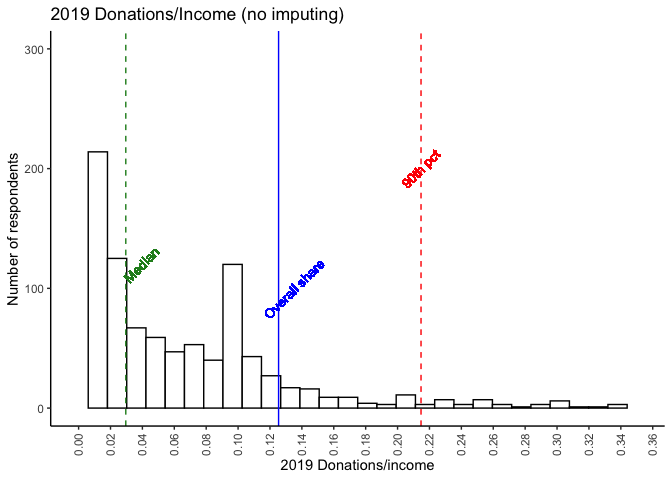##Todo -- Medium priority: mean is missing # todo -- low priority: make the above histogram bigger, it's smaller than the rest don_share_inc_19_hist_imp <- eas_20 %>% hist_plot(don_share_inc_19_imp_bc5k) + geom_vline_mean(tot_don/tot_inc_imp_bc, tgap=0.01, label = "Overall share") + geom_vline_med(eas_20$don_share_inc_19_imp_bc5k, tgap=0.005) +
geom_vline_90(eas_20$don_share_inc_19_imp_bc5k, tgap=0.005) + scale_x_set + labs(title="2019 Donations/Income (with imputing)", x="2019 Donations/income (with imputing)", y = "Number of respondents") + ylim(0, 300) don_share_inc_19_hist_imp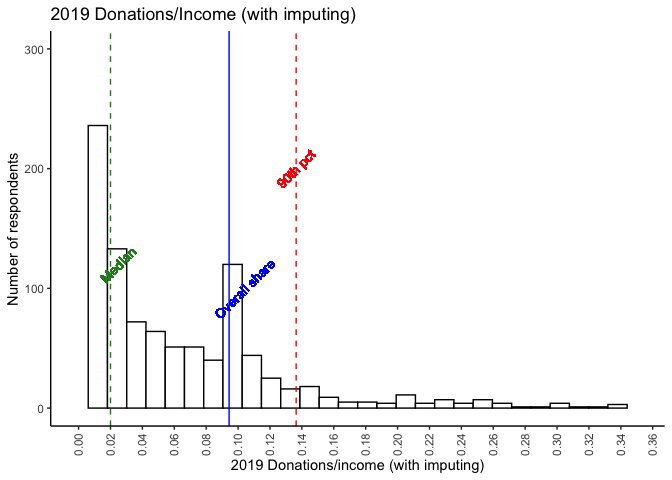#Todo -- Medium priority(@oska): convert to 'share of respondents', add cumulative plot don_share_inc_19_hist_imp %>% ggplotly() The noticeable spike at 10% likely reflects the GWWC pledge (we return to this further below). As noted above, 20% of EAs reported a donation at or above 10% of their (imputed) income in 2019. 36% reported an amount at or above 5%. #Donations and donation shares -- scatterplots by income and GWWC 'action' p_load(ggpubr) op_ax <- function(x) round(as.numeric(x), digits=2) scale_y_don <- scale_y_log10( name = "Donation amount (bottom-coded at$50)",
# labels = scales::dollar,
labels = scales::label_number_si(prefix = "$"), n.breaks = 10, limits = c(50, NA) ) don_income_gwwc_sp <- eas_all %>% filter(year==2020) %>% ggpubr::ggscatter( x = "income_c_imp_bc_k", y = "donation_usd_min50", color = "d_gwwc_ever", size = 0.8, xlab = "Income in$1k USD (imputed where missing or lt 5k)", repel = TRUE, palette = "jco", yscale = "log10", xscale = "log10", add = "loess", add.params = list(color = "black", fill = "lightgray"), conf.int = TRUE
) +
labs(title = "Donations by income (log scales)") +
scale_x_log10(name="Income in $1K USD (imputed if <5k/missing)", labels = op_ax, n.breaks=5, limits=(c(5,5000))) + labs(colour = "Mentioned taking GWWC pledge") + scale_y_don + theme(axis.text.x = element_text( angle = 90, vjust = 0.5, hjust = 1 )) don_income_gwwc_sp_gwwc <- eas_all %>% filter(year==2020) %>% ggplot(aes(x = income_c_imp_bc_k, y = donation_usd_min50, color = d_gwwc_ever)) + geom_point(size = 1, alpha = 0.7) + # draw the points geom_smooth(aes(method = 'loess', fill = d_gwwc_ever)) + # @Oska -- note I am using local smoothing here. scale_x_log10(name = "Income in$1K USD (imputed if below 5k/missing)", n.breaks = 5, limits = c(5, 5000)) +
scale_y_log10(
name = "Donation amount (bottom-coded at $50)", # labels = scales::dollar, labels = scales::label_number_si(prefix = "$"),
n.breaks = 10,
limits = c(50, NA)
) +
scale_color_discrete(name = "GWWC pledge") +
scale_fill_discrete(guide = "none") +
theme(axis.text.x = element_text( angle = 90, vjust = 0.5, hjust = 1 ),
legend.position = c(.87,.15),
legend.background = element_rect(fill=alpha('blue', 0.01)))

##Todo -- Medium priority - clean up the above a bit more...  get the axes better so that we can really see the 'large mass in the middle a bit better. Maybe slightly smaller dots and bolder smoothed lines, perhaps different colors for the CI shading for each
# - perhaps use geom_pointdensity with different shapes to indicate regions of "larger mass"

# #TODO -- Add some layer to better capture the masses *exactly at* 10pct

# REVIEW
# We should note that this doesn't include those who donate nothing due to the log scale (pseudo log scale is a bit weird here as well)
require(ggpointdensity)

don_share_income_by_X  <- eas_all %>%
filter(year==2020) %>%
mutate(income_c_imp_bc5k_k = income_c_imp_bc5k/1000) %>%
rowwise() %>%
mutate(don_share_inc_19_imp_bc5k = min(don_share_inc_19_imp_bc5k, 0.4)) %>%
ungroup() %>%
group_by(d_gwwc_ever_0) %>%
mutate(med_gwwc = median(don_share_inc_19_imp_bc5k, na.rm=TRUE)) %>%
ungroup() %>%
group_by(engage_high_n) %>%
mutate(med_eng = median(don_share_inc_19_imp_bc5k, na.rm=TRUE)) %>%
ggplot(aes(x = income_c_imp_bc5k_k, y = don_share_inc_19_imp_bc5k)) +
geom_smooth(method = "loess") +
#geom_hline_med(y) +
geom_hline(yintercept=0.1, linetype="dashed", size=0.5, color = "red") +
scale_y_continuous(labels = scales::label_percent(accuracy = 1L)) +
scale_x_log10(breaks = scales::log_breaks(n=7)) +
scale_color_viridis_c("density of respondents") +
xlab("Income in $1K USD (imputed if missing, bottom-code at 5k)") + theme(axis.title.x = element_text(size = 10)) + ylab("Donations/Income (top-code at 40%)") don_share_income_by_engage_sp <- don_share_income_by_X + geom_hline(aes(yintercept=med_eng), linetype="dashed", size=0.5, color = "blue") + facet_wrap(~engage_high_n, nrow=3) + ylab("Donations/Income (top-coded at 50%)") + labs(title="By 'High-engagement': 2019 'Don. shares of income' by income (w. imputing)") don_share_income_by_gwwc_sp <- don_share_income_by_X + geom_hline(aes(yintercept=med_gwwc), linetype="dashed", size=0.5, color = "blue") + facet_wrap(~d_gwwc_ever_0) + labs(title="By GWWC: 2019 'Don. share of income' by income (w/ imputing)") How do donations relate to income, and does this relationship differ between those who mention that they took the Giving What We Can (10%) pledge? We first simply plot reported donations against income, simply dividing individuals (points) by whether they mention having taken the GWWC pledge. don_income_gwwc_sp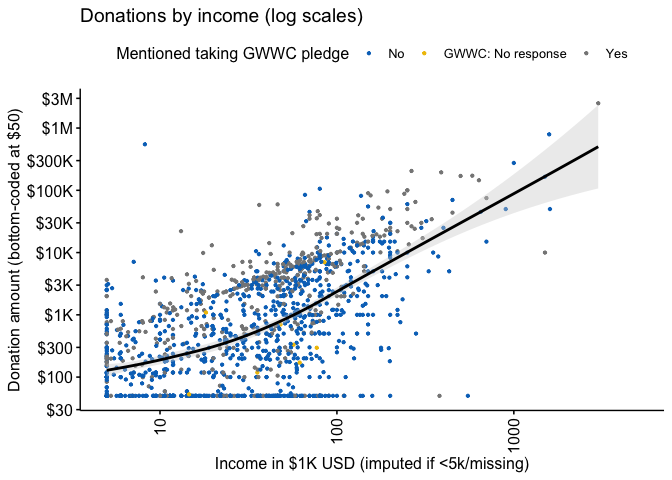We give a scatterplot of reported donations against income, faceted by GWWC pledge, with separate locally-smoothed conditional means (and 95% confidence intervals for these conditional means). (The figure below is for 2019 donations only.) don_income_gwwc_sp_gwwc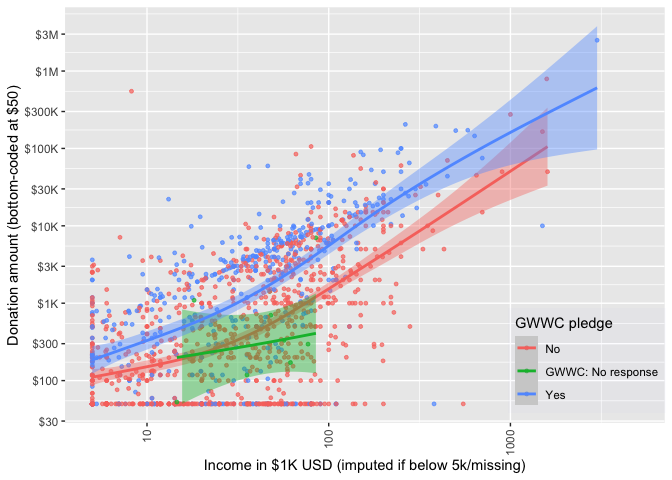Unsurprisingly, those with higher incomes, and those who took the GWWC pledge tend to report donating more. On average, the GWWC pledgers report giving more throughout the whole range of income, and the 95% confidence intervals are distinct for most of the range.*, ** * This agrees with what we reported in 2019: “In the EA Survey 2019 data, the median percentage of income donated by someone who had taken the GWWC Pledge was 8.87%, short of the 10% target, though there could be some noise around how respondents reported income and donations. Nevertheless, this of course could be influenced by GWWC Pledge takers being students, not employed or only recently having taken the Pledge. We addressed this question in more depth last year (link): GWWC members donate more than non-GWWC members, both absolutely and as a percentage of income but ~40% of self-reported GWWC members were not reporting donation data that is consistent with keeping their pledge, a trend most likely to be the result of attrition over time.” ** Note that the smaller group who did not respond to the GWWC pledge prompt but did provide a donation response seems to resemble the non-pledgers. We thus lump these groups together in the subsequent analysis. Next we plot donations as shares of income against income for non-GWWC pledgers (combined with non-responders) and GWWC pledgers. The median for each group is given by the dashed blue line, and the dashed red line represents 10 percent of income. don_share_income_by_gwwc_sp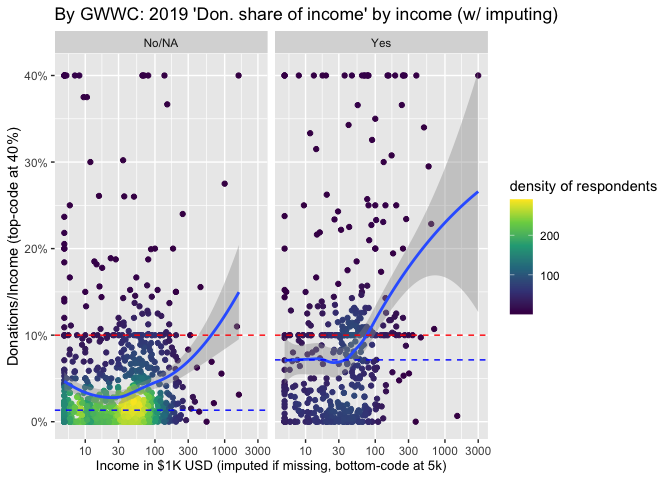The relationship between income and ‘share of income donated’ dips down for the lowest incomes, but for the mass of ‘substantial donors’ the curve is fairly flat, and then seems to increase at higher incomes. As expected, GWWC pledgers tend to donate closer to 10% of income than do the rest. In each year substantially larger shares of those who report having made a GWWC pledge report donating 10% or more. Below, we tabulate this by donation year and by ’whether they report having ever made a GWWC pledge, for individuals who report income over 5000 USD and who report zero or positive donations: ( tab_don_by_year_pledge <- eas_all %>% filter(!is.na(d_don_10pct_bc5k) & year>=2015) %>% mutate(Survey year = year, d_don_plan_10pct = as.numeric(donation_plan_usd/income_c_imp_bc5k >=0.1), d_don_plan_10pct = if_else(year<2018, NaN, d_don_plan_10pct)) %>% group_by(d_gwwc_ever_0, Survey year) %>% summarise(n = n(), "Donated 10% of income" = mean(d_don_10pct_bc5k), "Donated 10% of income (plan)" = mean(d_don_plan_10pct, na.rm=TRUE) ) %>% rename("Ever GWWC pledge" = d_gwwc_ever_0) %>% adorn_rounding(digits = 2) %>% kable(caption = "GWWC pledgers: Don. 10%+ of income by survey year (exclusions: see text)", label=TRUE) %>% .kable_styling() ) Table 4.3: GWWC pledgers: Don. 10%+ of income by survey year (exclusions: see text) Ever GWWC pledge Survey year n Donated 10% of income Donated 10% of income (plan) No/NA 2015 819 0.13 NaN No/NA 2017 673 0.16 NaN No/NA 2018 1221 0.13 0.19 No/NA 2019 1125 0.12 0.16 No/NA 2020 960 0.11 0.19 Yes 2015 352 0.36 NaN Yes 2017 354 0.35 NaN Yes 2018 668 0.40 0.53 Yes 2019 579 0.37 0.48 Yes 2020 463 0.40 0.48 Among those who report having ever taken a GWWC pledge (and who report donations, and excluding those reporting incomes below 5000 USD), less than half report donating 10% in the past year. However, this may be an underestimate, as some people are reporting having pledged for this/next year, while donation reports are for the previous year.* * Furthermore, this does not tell us that people are failing to meet an active pledge. The question asks about having ever taken the GWWC pledge’; some of these people might have ended their pledge at some point. Our 2018 post report found a rate slightly higher than 50%.** This is closer to the above figure for ‘plan to donate in the current year,’ which hovers around 50%.*** ** The rates we report may also be lower than those reported in the 2018 post because here we exclude those earning less than 5000 USD. *** In the online appendix (web link we also plot donations by income by self-reported level of engagement (1-3 versus 4-5). Unsurprisingly, those who report greater engagement tend to donate more. ### 4.3.2 Employment and student status We present income and donation statistics for those “statuses” with more than 50 respondents in the forest plot below (a full table of statistics for each group can be found in the bookdown appendix).* In each of the forest plots in this subsection, the blue line presents a simple linear best-fit of these points, and the red line represents a 10% donation rate. * In stratifying donation and income statistics by employment/student status we exclude those who gave no information on this question (or who answered that they prefer not to answer). (These nonresponses make up 20.4% of the sample). se <- function(x) sqrt(var(x)/length(x)) sumstatvec <- c("{median}", "{p10}-{p90}", "{mean} [{se}] ({sd})") doninclabs <- list(income_k_c ~ "Income in$1000 USD",
donation_2019_c ~ "2019 donation (in USD)",
donation_2020_c ~ "2020 planned donation")
don_inc_by_student <-
eas_20 %>%
group_by(status_) %>%
mutate(
status_ = as.character(status_),
large_group = case_when(
n()<50 ~ "Other",
TRUE ~ status_)
) %>%
ungroup() %>%
dplyr::select(income_k_c, donation_2019_c, donation_2020_c, large_group) %>%
tbl_summary(by = large_group,
type = c(all_continuous()) ~ "continuous2",
statistic = list(all_continuous() ~ sumstatvec),
label = doninclabs,
missing = c("no") ) %>%
bold_labels() %>%

#TODO: High -- fix the column labels
#todo (low) -- we use this several times and it's a good format; let's functionalise it
#Todo (medium): Bootstrapping the SE of the median would be nice, see, e.g., https://clayford.github.io/dwir/dwr_12_generating_data.html
library(ggrepel)
#
# 1.summarize donation and income (mean and 95pct CI for each) by status_
# 2. plot median (and mean) donation by income for each group (income lowest to highest)
# 3. fit a line/curve of donation by income for each group (do for ) -- replace with the regression line based on the population not the groups
# 4. Add error bars (for donations, not income) -- hard to do for median, though

#TODO -- High Priority: Make this nice in the ways discussed (@oska it seems you have already started this)

# why are the error bars not surrounding the point?
# make it pretty (use your judgment), fix labels, add median colored dot,

(
don_inc_status_plot <- eas_20 %>%
mutate(
status_ = str_replace_all(
status_, c("_" = " ")
)
) %>%
grp_sum(income_c_imp_bc5k, donation_2019_c, status_) %>%
plot_grp(status_, labsize=3, fp=0.3, force=5, mo=20, bp=1.5,
arrow = arrow(length = unit(0.02, "npc"))
) +
xlab("Mean income in USD (imputed if < 5k/missing)") +
ylab("Mean donations, 95% CIs") +
scale_y_continuous(limits=c(-10000, 30000),  oob = scales::squish)
)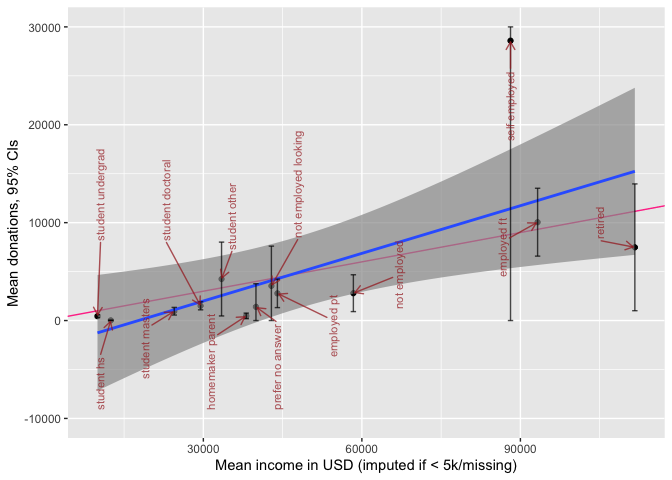# Todo (low): Plot regression line for full pop
# Todo: HIGH -- get this to look nicer, label it better, add better axis breaks (every 5k for donation, every 20k for income)
#Todo (Medium) -- add plots for the medians
#Todo

Donations generally track income by this aggregation, with some groups possibly ‘under-performing’ or ‘over-performing’; we return to this in our descriptive modeling.*

*Note that thus is reporting means and not medians. The ‘self-employed’ group clearly reflects outliers, and it’s upper CI is truncated at 30000 to save space.

### 4.3.3 Donations by country

Donations and income by country

We report similar income and donation statistics for all countries with more than 50 respondents:

(
don_income_by_ctry <-
eas_20 %>%
dplyr::select(income_k_c, donation_2019_c, donation_2020_c, country_big) %>%
tbl_summary( by = country_big,
sort = all_categorical() ~ "frequency", #reverse this ordering or maybe reverse sort by average income
type = c(all_continuous()) ~ "continuous2",
statistic = list(all_continuous() ~ sumstatvec),
label = doninclabs,
missing = c("no")
) %>%
bold_labels() %>%
)
Income in $1000 USD 1,384 Median 33 36 27 13 17 15 22 36 50 10%-90% 1-130 7-109 3-86 0-42 2-70 0-64 0-83 3-82 2-192 Mean [se] (SD) 60  (136) 49  (46) 41  (42) 18  (17) 28  (29) 31  (38) 37  (44) 69  (243) 85  (146) 2019 donation (in USD) 1,397 Median 533 872 231 178 355 237 327 660 1,000 10%-90% 0-9,577 0-7,271 0-6,948 0-3,551 0-4,971 0-2,367 0-6,450 0-6,600 0-16,180 Mean [se] (SD) 7,348 [1,956] (73,113) 3,647  (8,411) 2,753  (6,769) 1,178  (1,919) 1,582  (2,850) 925  (1,536) 4,069 [1,777] (31,444) 21,019 [12,693] (182,619) 7,211  (20,215) 2020 planned donation 1,377 Median 1,000 1,636 761 473 947 395 592 1,320 2,000 10%-90% 0-12,020 11-12,360 0-8,676 0-4,660 17-5,823 0-4,438 0-7,000 0-7,867 0-21,000 Mean [se] (SD) 9,831 [2,001] (74,249) 4,852  (8,404) 2,909  (6,142) 2,026  (3,450) 2,216  (3,692) 1,358  (1,901) 3,515  (10,654) 24,930 [11,970] (170,546) 12,033 [2,345] (54,382) 1 c("Median", "10%-90%", "Mean [se] (SD)") #todo (medium?): make a stem-leaf thing here #todo (High): add *medians* to the above  # don_inc_status_plot <- eas_20 %>% # dplyr::select(status_, donation_2019_c, income_k_c) %>% # group_by(status_) %>% # drop_na(status_, donation_2019_c, income_k_c) %>% # summarise(across(c(donation_2019_c, income_k_c), # list(mean=mean, # median=median, # se = ~sd(.x)/sqrt(length(.x))))) %>% # group_by(status_) %>% p_load(ggimage) country_codes <- tibble(country = c("Australia", "Canada", "France", "Germany", "Netherlands", "Other", "United Kingdom", "USA"), code = c("ac", "ca", "fr", "de", "nl", "yt", "gb", "us")) ( don_inc_country_plot <- eas_20 %>% grp_sum(income_c_imp_bc5k, donation_2019_c, country_big) %>% left_join(., country_codes, by = c("country_big" = "country")) %>% plot_grp(country_big) + xlab("Mean income in USD (imputed if <5k/missing)") + ylab("Mean donations, CIs") + scale_y_continuous(limits=c(-3000, 30000), oob = scales::squish) )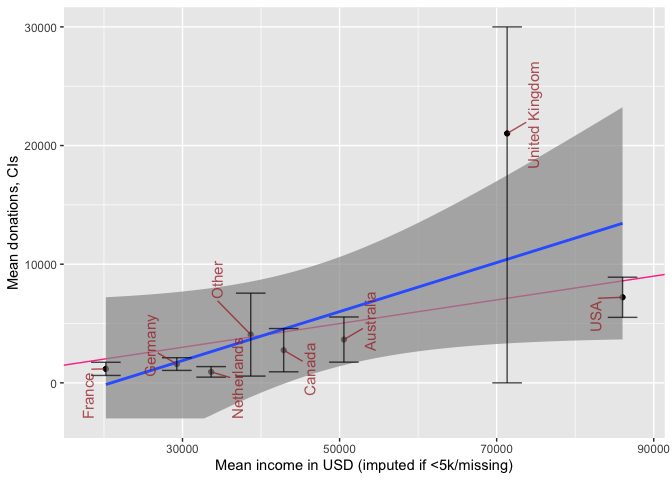#+ggimage::geom_flag() Above, we plot donations and income by country of residence for the countries with the largest number of EA respondents. We fit a simple best-fit (least-squares) line in blue, and add a red line depicting a 10% donation rate. Again, donations generally track income, with some under and over-performers (see later modeling). The UK clearly contains some notable donation outliers, leading to very large confidence intervals for the UK mean (truncated above at 30000 USD). plot_box_pt_viol <- function(df, yvar, groupvar, notch=TRUE) { df %>% dplyr::select({{yvar}}, {{groupvar}}) %>% ggplot() + aes({{groupvar}}, {{yvar}}) + geom_point(size = 0.30, colour = "grey", position = position_jitter(seed = 42, width = 0.3, height = 0.01)) + geom_boxplot(alpha=0.7, notch=notch, color="black") + geom_violin(alpha=0.4, color = "pink") + scatter_theme + scale_y_log10() } ( don_by_country_viol_20 <- eas_20 %>% plot_box_pt_viol(donation_2019_c, country_big, notch=TRUE) + labs(title = "Donation amounts by country (2019)") ) ( don_by_country_viol_all <- eas_all %>% plot_box_pt_viol(donation_usd, where_live_cat, notch=TRUE) + labs(title = "Donation amounts by country grouping (2013-2019)") ) ( don_by_yr_viol_all <- eas_all %>% mutate(year=as.factor(year)) %>% plot_box_pt_viol(donation_usd, where_live_cat, year) + ggplot() + labs(title = "Donation amounts by year") ) ### Donations, age and years in EA Next, we consider how donations may increase or decrease with ‘time-in-EA’ (i.e., ‘tenure’). As discussed in other posts and bookdown chapters, this may be reflecting differences in who stays in EA (and continues responding to the survey) as much as it reflects how people themselves change from year to year. Below, we plot donations by tenure, breaking this down by age groups. don_by_tenure_facet_age <- eas_all %>% filter(year==2020) %>% filter(!is.na(age_ranges)) %>% ggplot() + aes(x = tenure, y = donation_usd_min50) + geom_point(size = 0.15, colour = "#0c4c8a", position = position_jitter(seed = 42, width = 0.1, height = 0.001)) + geom_smooth(span = 0.75) + scatter_theme + facet_grid(vars(), vars(age_ranges), scales = "free") + labs(title = "2019 donation by time in EA", subtitle = "Faceted by Age ranges") + labs(x = get_label(eas_20$tenure)) +
don_by_tenure_facet_age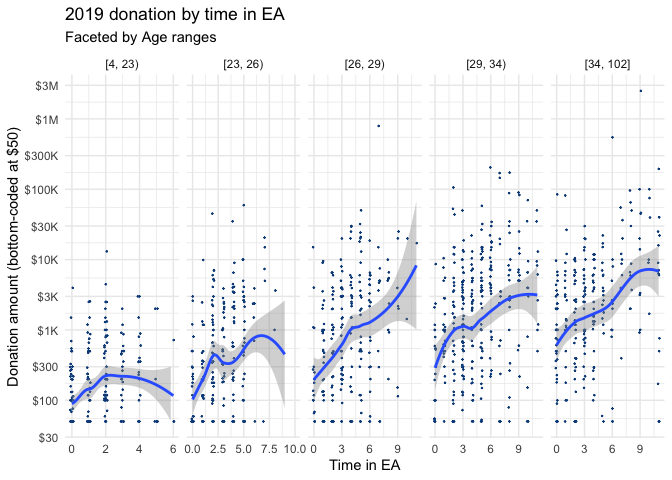don_by_tenure_facet_age %>% ggplotly()